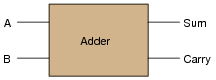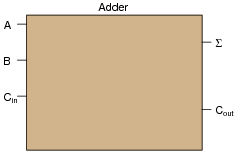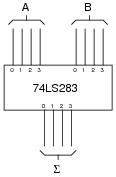# Binary Math Circuits

## Digital Circuits

• #### Question 1• #### Question 2

 Don’t just sit there! Build something!!

Learning to analyze digital circuits requires much study and practice. Typically, students practice by working through lots of sample problems and checking their answers against those provided by the textbook or the instructor. While this is good, there is a much better way.

You will learn much more by actually building and analyzing real circuits, letting your test equipment provide the “answers” instead of a book or another person. For successful circuit-building exercises, follow these steps:

1. Draw the schematic diagram for the digital circuit to be analyzed.
2. Carefully build this circuit on a breadboard or other convenient medium.
3. Check the accuracy of the circuit’s construction, following each wire to each connection point, and verifying these elements one-by-one on the diagram.
4. Analyze the circuit, determining all output logic states for given input conditions.
5. Carefully measure those logic states, to verify the accuracy of your analysis.
6. If there are any errors, carefully check your circuit’s construction against the diagram, then carefully re-analyze the circuit and re-measure.

Always be sure that the power supply voltage levels are within specification for the logic circuits you plan to use. If TTL, the power supply must be a 5-volt regulated supply, adjusted to a value as close to 5.0 volts DC as possible.

One way you can save time and reduce the possibility of error is to begin with a very simple circuit and incrementally add components to increase its complexity after each analysis, rather than building a whole new circuit for each practice problem. Another time-saving technique is to re-use the same components in a variety of different circuit configurations. This way, you won’t have to measure any component’s value more than once.

• #### Question 3

Identify each of these logic gates by name, and complete their respective truth tables:• #### Question 4

Design a circuit that adds two binary bits together. This circuit will have two inputs (A and B) and two outputs (Sum and Carry):Begin the design process by drawing a truth table for the circuit, then determining the necessary gate circuitry to fulfill each output function.

Why is this circuit referred to as a half adder? How would a full adder circuit differ from this?

• #### Question 5

Design a circuit that adds two binary bits and a “Carry in” (Cin) bit together, producing a “Sum” (Σ) and a “Carry out” (Cout) output:Begin the design process by drawing a truth table for the circuit, writing a boolean SOP expression for each output, then determining the necessary gate circuitry to fulfill each output function.

• #### Question 6

Explain the difference between a ripple adder and a look-ahead adder. What does the term “ripple” mean in this context? Why is “ripple” potentially a bad thing for a digital adder circuit?

• #### Question 7

Compare the following two circuits, the first one being a digital adder and the second one being an analog summer:These two circuits perform the same mathematical function, yet the manners in which they perform this function are quite different. Compare and contrast the digital adder and the analog summer circuits shown here, citing any advantages or disadvantages of each.

• #### Question 8

Explain the purpose of a magnitude comparator IC such as the 74LS85. What function, or functions, does it perform?

• #### Question 9

Research the datasheet of an integrated arithmetic logic unit such as the 74AS181, and determine how its various modes of operation (addition, subtraction, comparison) are selected.

• #### Question 10

An arithmetic trick often used when working with the metric system is multiplication-by-ten and division-by-ten via shifting of the decimal point. A similar “trick” may be applied to binary numbers, with similar results.

Determine what sort of multiplication or division is accomplished when the “binary point” is shifted in a binary number. Research the datasheet of an arithmetic logic unit (ALU) circuit to see if and how this function is implemented.

• #### Question 11

Explain the meaning of the digital lines A, B, F, and S in the following schematic diagram: